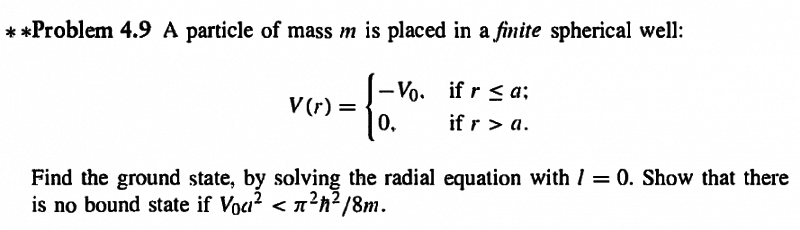# Bound states in finite spherical well

Gold Member

## Homework Statement## The Attempt at a Solution

for ##r \le a## and ##l = 0##, the radial equation is

$$- \frac {\hbar^{2}}{2m} \frac {d^{2}u}{dr^{2}} - V_{0} = Eu$$
$$- \frac {\hbar^{2}}{2m} \frac {d^{2}u}{dr^{2}} - [V_{0} + E]u = 0$$
call ##k^{2} = \frac {2m}{\hbar^{2}}[V_{0} + E]##
The solution to this differential equation comes out to be
$$u(r) = A sin(kr) + B cos(kr)$$
Since ##u = R(r)r##, as ##r \rightarrow 0##, then ##\frac {cos(kr)}{r} \rightarrow \infty##, therefore ##B = 0##
Leaving ##u(r) = Asin(kr)##

For ##r > a##
$$- \frac {\hbar^{2}}{2m} \frac {d^{2}u}{dr^{2}} = Eu$$
##\kappa^{2} = - \frac {2mE}{\hbar^{2}}##
therefore, ##u(r) = Ce^{-\kappa r} + De^{\kappa r}##
As ##r \rightarrow \infty##, then the second term blows up, so ##D = 0##

At the ##r = a##, the ##u(r)## must be the same for both sides. Therefore,
##A sin(ka) = Ce^{- \kappa a}##
Also, the function must be continuous, so the derivative must be equal at ##r = a##
## kA cos(ka) = - \kappa C e^{-\kappa a}##
Dividing through,
$$\frac {1}{k} tan(ka) = - \frac {1}{\kappa}$$

From here, how am I supposed to find the bound states? I have always been confused about bound and unbound states.

Hi Maylis,
Try to expand tan(ka)
Note that the ground state have E~0
but no zero, we can give it a great approximation !

PeroK
Homework Helper
Gold Member
2020 Award

## Homework Statement

View attachment 83427

## The Attempt at a Solution

for ##r \le a## and ##l = 0##, the radial equation is

$$- \frac {\hbar^{2}}{2m} \frac {d^{2}u}{dr^{2}} - V_{0} = Eu$$
$$- \frac {\hbar^{2}}{2m} \frac {d^{2}u}{dr^{2}} - [V_{0} + E]u = 0$$
call ##k^{2} = \frac {2m}{\hbar^{2}}[V_{0} + E]##
The solution to this differential equation comes out to be
$$u(r) = A sin(kr) + B cos(kr)$$
Since ##u = R(r)r##, as ##r \rightarrow 0##, then ##\frac {cos(kr)}{r} \rightarrow \infty##, therefore ##B = 0##
Leaving ##u(r) = Asin(kr)##

For ##r > a##
$$- \frac {\hbar^{2}}{2m} \frac {d^{2}u}{dr^{2}} = Eu$$
##\kappa^{2} = - \frac {2mE}{\hbar^{2}}##
therefore, ##u(r) = Ce^{-\kappa r} + De^{\kappa r}##
As ##r \rightarrow \infty##, then the second term blows up, so ##D = 0##

At the ##r = a##, the ##u(r)## must be the same for both sides. Therefore,
##A sin(ka) = Ce^{- \kappa a}##
Also, the function must be continuous, so the derivative must be equal at ##r = a##
## kA cos(ka) = - \kappa C e^{-\kappa a}##
Dividing through,
$$\frac {1}{k} tan(ka) = - \frac {1}{\kappa}$$

From here, how am I supposed to find the bound states? I have always been confused about bound and unbound states.

Bound states are defined by E < 0, which you assumed to get the solution outside the well. So, this is the solution for bound states you have.

To solve the transcendental equation in ##tan(ka)## you need a bit of jiggery-pokery. See the earlier chapter on the 1D finite well for how it's done. Let ##z = ka## etc.